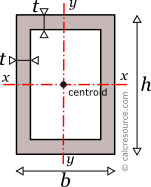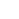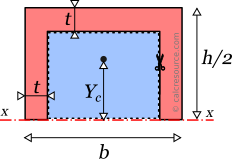## Rectangular Tube Section Calculator

This tool calculates the properties of a rectangular tube (also called rectangular hollow cross-section or RHS). Enter the shape dimensions b, h and t below. The calculated results will have the same units as your input. Please use consistent units for any input.

 b = h = t = Geometric properties: Area = Outer Per. = Inner Per. = Total Per. = Properties related to x-x axis: Ix = Sx = Zx = Rgx = ADVERTISEMENT Properties related to y-y axis: Iy = Sy = Zy = Rgy = Other properties: Iz =## Definitions

### Geometry

The area , the outer perimeter and inner perimeter of a rectangular tube section, can be found with the next formulas:

where b, h the external sides of the rectangular tube section and , the respective internal ones. The latter are:

• the internal width
• the internal height

where , the thickness of the walls.

### Moment of Inertia

The moment of inertia (second moment of area) of a rectangular tube section, in respect to an axis x passing through its centroid, and being parallel to its base b, can be found by the following expression:

where b is the section width, and specifically the dimension parallel to the axis, and h is the section height (more specifically, the dimension perpendicular to the axis), , the internal width and the internal height. Because the moment of inertia of a solid rectangle is , the moment of inertia of the rectangular tube, can be seen as a difference between two rectangular areas: an external rectangle with dimensions , , minus an internal one, with dimensions , .

Similarly, the moment of inertia (second moment of area) of a rectangular tube, around a centroidal axis y, perpendicular to base b, can be found, by an equation similar to the previous one but with interchanged the width and height dimensions:

The moment of inertia (second moment or area) is used in beam theory to describe the rigidity of a beam against flexural bending. The bending moment M applied to a cross-section is typically related to the moment of inertia of the cross-section with the following equation:

where E is the Young's modulus, a property of the material, and the curvature of the beam due to the applied load. Therefore, it can be seen from the former equation, that when a certain bending moment M is applied to a beam cross-section, the developed curvature is reversely proportional to the moment of inertia I.

The polar moment of inertia, describes the rigidity of a cross-section against torsional moment, likewise the planar moments of inertia described above, are related to flexural bending. The calculation of the polar moment of inertia , around an axis z (perpendicular to the section), can be done with the Perpendicular Axes Theorem:

where, and , are the moments of inertia around axes x and y that are mutually perpendicular with z-z and meet at a common origin.

The dimensions of moment of inertia are .

### Elastic modulus

The elastic section modulus of any cross section, around its centroidal axis x, describes the response of the section under elastic flexural bending, around the same axis. It is defined as:

where , the moment of inertia of the section around x axis and Y the distance from centroid, of a section fiber, parallel to the same axis. Typically the more distant fiber is of interest. If a cross-section is symmetric (the rectangular tube is), around an axis (e.g. centroidal x) and its dimension perpendicular to this axis is h, then Y=h/2 and the above formula becomes:

Similarly, for the section modulus , around y axis, which happens to be an axis of symmetry too, the above definitions are written as:

If a bending moment is applied around axis x-x, the section will respond with normal stresses, varying linearly with the distance from the neutral axis (which under elastic regime coincides to the centroidal x axis). Over neutral axis, the stresses are be definition zero. Absolute maximum , will occur at the most distant fiber, with magnitude given by the formula:

From the last equation, the section modulus can be considered for flexural bending, a property analogous to cross-sectional A, for axial loading. For the latter, the normal stress is F/A.

The dimensions of section modulus are .

### Plastic modulus

The plastic section modulus is similar to the elastic one, but defined with the assumption of full plastic yielding of the cross section, due to flexural bending. In that case the whole section is divided in two parts, one in tension and one in compression, each under uniform stress field. For materials with equal tensile and compressive yield stresses, this leads to the division of the section into two equal areas, in tension and in compression, separated by the neutral axis. This is a result of equilibrium of internal forces in the cross-section, under plastic bending. Indeed, the compressive force would be , assuming the yield stress is equal to , in compression, and that the material over the entire compressive area has yielded (thus the stresses are equal to everywhere). Similarly, the tensile force would be , using the same assumptions. Enforcing equilibrium:

The axis is called plastic neutral axis, and for non-symmetric sections, is not the same with the elastic neutral axis (which again is the centroidal one).The plastic neutral axis divides the cross section in two equal areas, provided the material features equal tensile and compressive yield strength.

#### Around x axis

The plastic section modulus is given by the general formula (assuming bending around x axis):

where , the distance of the centroid of the compressive area , from the plastic neutral axis, and , the respective distance of the centroid of the tensile area .

For the case of a rectangular tube cross-section, the plastic neutral axis passes through centroid, dividing the whole area into two equal parts. Taking advantage of symmetry, it is: . Finding these centroids, is straightforward. We will consider the part above the neutral axis (assumed in compression). The centroid of this part is located at a distance from the plastic neutral axis. It is convenient to assume that the whole part, is equivalent to the difference between an outer rectangle, having dimensions, b and h/2 minus an inner rectangle, with dimensions b-2t and h/2-t. The centroid of the whole part is then found, making the static moment of inertia (first moment of area), of the whole part, equal to the difference of its two rectangular areas. Remember that the examined part has half the total section area:

where:

• , the inner width of the tube cross-section, and
• , the inner height of the tube cross-sectionUpper half part of the rectangular tube

Taking into consideration that (due to symmetry) and given that , the plastic section modulus, of a rectangular tube, around x axis, is found:

We could have reached to the same formula, a lot faster if we had as given the plastic modulus of a rectangular area, with dimensions b and h, around a centroidal axis, perpendicular to h. This is:

The whole rectangular tube could then be considered as the difference between the outer rectangle, with dimensions and and the inner rectangle with dimensions and , as illustrated in the following figure. Both rectangles share the same centroidal x axis (otherwise we could not proceed). With this consideration, the above formula is derived naturally, as the difference of the plastic moduli between these two rectangles.

#### Around y axis

Following a similar procedure, the plastic section modulus, around y axis, is found by the following formula:

Notice, that the last formula is similar to the one for the plastic modulus , but with the height and width dimensions interchanged.

### Radius of gyration

Radius of gyration of a cross-section, relative to an axis, is given by the formula:

where I the moment of inertia of the cross-section around the same axis and A its area. The dimensions of radius of gyration are . It describes how far from centroid the area is distributed. Small radius indicates a more compact cross-section. Circle is the shape with minimum radius of gyration, compared to any other section with the same area A. The rectangular tube, however, typically, features considerably higher radius, since its section area is distributed at a distance from the centroid.

### Rectangular tube section formulas

The following table, lists the main formulas, discussed in this article, for the mechanical properties of the rectangular tube section (also called rectangular hollow section or RHS).

### Related pages

Properties of a circular tube section
Properties of a Circular section
Properties of a Rounded Rectangle section
Moment of Inertia of a Rectangle
All Cross Section tools

Liked this page? Share it with friends!